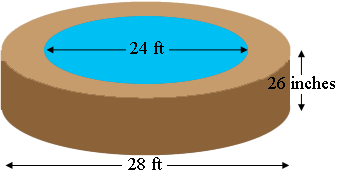SEARCH HOMEMath Central Quandaries & QueriesQuestion from steve: How sand is needed to back fill a trench around a 24ft dia. pool that is 26" deep by 2ft wide. Making the outside dia. 26ft. Thank YouHi Steve,

My diagram is not to scale and I also changed one of the dimensions. If the pool has a diameter of 24 feet and the trench is 2 feet wide then the outside diameter is 28 feet.I would first convert the diameters to inches.

Use our volume calculator to find the volume of a cylinder with diameter 28 feet (converted to inches) and depth 26 inches. Use the volume calculator again to find the volume of a cylinder with diameter 24 feet (converted to inches) and depth 26 inches.The difference is the volume of sand you need.

PennyMath Central is supported by the University of Regina and The Pacific Institute for the Mathematical Sciences.